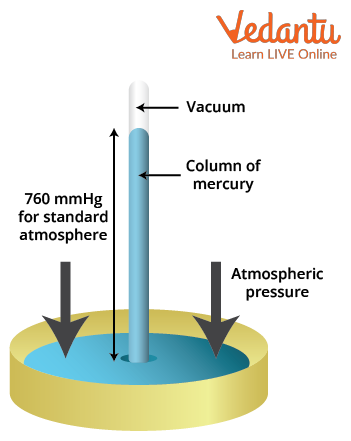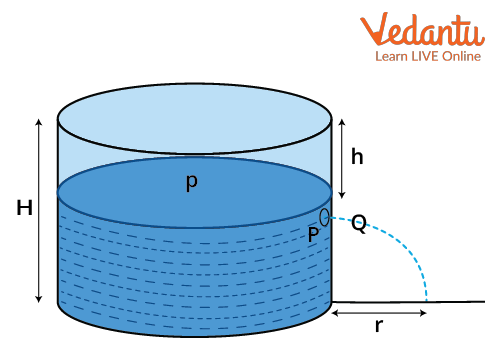Courses
Courses for Kids
Free study material
Free LIVE classes
More

# Torricelli’s Theorem, Derivation and Solved Examples for JEELIVE
Join Vedantu’s FREE Mastercalss

## Introduction to Torricelli’s Theorem

Torricelli's theorem was invented by the scientist, Evangelista Torricelli in the year 1643 and this theorem has various aspects of applications in physics. This law can be seen in many aspects of our daily life. Torricelli's theorem mainly tells the relationship between the liquid velocity and the height h.

Torricelli's theorem is a concept which comes into play when we are studying the fluids placed in a container. Let us assume there is a hole in a liquid container consisting of water in it at the depth of h from the top surface of the cylindrical container. Eventually, water starts flowing out of the container through that hole with a velocity and hits the ground. This is where Torricelli's theorem is used. We call the process in which the water flows out of the container through a hole Efflux.

Let us understand what Torricelli's law means and let’s state and prove Torricelli's theorem through this article.

## What is Torricelli’s Theorem?

According to the Torricelli's theorem, the velocity that is attained by water when it starts flowing out of a hole to the ground due to the force of gravity would be equal to the velocity of water that it attains when allowed to fall freely through the height from the top liquid surface to the hole. This is also often termed Torricelli's equation or Torricelli's law in fluids.

We could simplify it as the speed of the water during the efflux from height h is the same as the velocity that water gets when it is allowed to free-fall due to the gravitational force under the same distance h. This theorem is independent of the direction of flow of water at the opening hole and this theorem should be satisfied even if the hole is in any other direction be it upwards or downwards. Let us understand how Torricelli’s theorem is derived and proven.

## Torricelli’s Experiment with Barometer

Evangelista Torricelli, in his experiment, used a simple barometer which is filled with mercury to the three-fourths of the tube to measure the pressure of the air and the air bubbles are removed. Now the pure mercury is filled again into the tube to its full and it is placed inverted in a dish which is also filled with mercury. This causes the mercury in the tube to come down till we can see the difference between mercury on the surface and in the tube is 760 mm.Image: Torricelli’s Experiment with Barometer and Mercury

This is the setup used for the experiment. Let us see how Torricelli derived the velocity efflux equation through this experiment.

## Velocity Efflux Derivation

Let us imagine a container containing water filled in it is placed on the ground and its height is H. Let us open a hole in the side part of the container tank at a height h from the top liquid surface of the container as shown in the figure below.Image: A hole in a container at the height h from the surface

Gradually, water keeps coming out from the hole with a velocity, v. Let us assume a point inside the container just beside the hole as P and a point outside the container as Q which is also beside the hole. Let's start by applying Bernoulli's theorem at those points.

Bernoulli's theorem states that in an incompressible and non-viscous liquid, the sum of pressure energy, kinetic energy and the gravitational potential energy per unit mass remains constant. This is a law which strives to conserve energy in fluid dynamics. This tells us that the velocity of the fluid occurs concurrently with the decrease in the fluid’s potential energy.

The following is the equation which describes Bernoulli's theorem clearly.

$P_{f}+\dfrac{1}{2} \rho v^{2}=\text { constant }$

Here,  $\dfrac{1}{2} \rho v^{2}$ is the kinetic energy of the fluid that is in motion and Pf is the fluid pressure which is equal to the sum of the fluid’s potential energy and the atmospheric pressure.

$P_{f}=P_{a t m}+\rho g h$

Generally, this comes from the definition that the total pressure of a fluid is equal to the sum of the atmospheric pressure and the pressure on the fluid due to gravity. Atmospheric pressure is the weight of the fluid per the area of the atmosphere above the fluid whereas the pressure due to gravity is the weight of the fluid per the area of the fluid.

Therefore, Bernoulli's theorem can be written as

$P_{a t m}+\rho g h+\dfrac{1}{2} \rho v^{2}=\text { Constant }$

Here $\rho$ is the density of the fluid, g is the acceleration due to gravity and h is the height of the point from the surface of the liquid or its depth from the top liquid surface, v is the velocity of the fluid at that point. Let us apply Bernoulli’s Theorem at Points P and Q and equalise them as the energy should be conserved.

At Point P, As the area of the cross-section P is occupied by a large mass of water and the velocity of water flow is very much less compared to that of velocity at which water is leaving the hole. So the velocity of the fluid is almost equal to zero and so the kinetic energy can be neglected.

$P_{a t m}+\rho g h=\text { constant }$

And at point Q, there is no fluid’s potential energy due to gravitational force.

$P_{a t m}+\dfrac{1}{2} \rho v^{2}=\text { Constant }$

I.e., $P_{a t m}+\rho g h=P_{a t m}+\dfrac{1}{2} \rho v^{2}$

$2 \rho g h=\rho v^{2}$

Therefore, v2 = 2gh

And $v=\sqrt{2gh}$

This is the equation that is termed Torricelli's equation and it is widely used in fluid dynamics. So the velocity with which the liquid is coming out of the hole is called the efflux velocity and it is given by the above equation.

Let us solve some problems with this theorem.

## Solved Examples on Torricelli’s Theorem

Example 1: A tank of height 100 metres is filled with water and there is a hole at a point which is 80 metres above the ground. Find the velocity of the fluid leaving the hole if the acceleration due to gravity is $10~\dfrac{m}{s^{2}}$ .

Ans:  Given that, H = 100m, h = 100 - 80 = 20m and $g = 10~\dfrac{m}{s^2}$

Therefore, according to the velocity efflux equation in torricelli’s theorem, we can find the velocity by the equation,

$v=\sqrt{2 g h}=\sqrt{2(10)(20)}=\sqrt{400}=20 \dfrac{\mathrm{m}}{\mathrm{s}}$

Therefore, the velocity of the water leaving the hole is 20 metres per second.

Example  2: A container whose height is 200 metres is filled with water and a hole is placed at a height h from the surface of the liquid. The velocity of the water leaving the hole is 40 m/s. Find the value of h if the acceleration due to gravity is taken as $10~\dfrac{m}{s^2}$.

Ans:  Given that, H = 200m, v = 40m/s and $a = 10~\dfrac{m}{s^2}$

Through the torricelli’s equation,

\begin{align} &v=\sqrt{2 g h} \\ &40=\sqrt{2(10) h} \\ &40^{2}=20 h \\ &h=80 m \end{align}

Therefore, the height of the hole from the top of the liquid surface is 80 metres.

## Conclusion

Torricelli's theorem is mainly based on Bernoulli's principle which states that the whole mechanical energy of a fluid which is in motion includes the fluid’s gravitational potential energy, the kinetic energy of the fluid in motion and the energy regarding the pressure of the fluid would remain constant. This is what we have applied at two points inside and outside the container at the hole section and we have derived the equation for Torricelli's Law. We learnt how to state and prove Torricelli's theorem through this article.

Last updated date: 02nd Oct 2023
Total views: 3.3k
Views today: 0.03k

## FAQs on Torricelli’s Theorem, Derivation and Solved Examples for JEE

1. What does Torricelli’s Experiment tell about the atmospheric pressure?

Atmosphere prominently exerts pressure on the mercury that is taken in a dish in Torricelli's experiment. This pressure eventually starts acting upwards and affects the mercury level in the tube. If there is no atmospheric pressure acting on the mercury, then the mercury present in the tube will fall down due to the force of gravity. Therefore, atmospheric pressure is equal to the pressure of the mercury present in the tube. The height of the liquid mercury can be used to detect the pressure due to the atmosphere on the mercury level.

2. What are the applications of Torricelli’s law in our daily lives?

This theorem has many applications practically in a human’s life. This describes the relationship between the velocity at which the fluid is leaving the container and the height from which the fluid’s efflux is made on the container. This principle is used in the water reservoirs where the height should be calculated from which the water must be left in the river. We can see this method even in drums or tanks that contain a huge amount of water. This is also used in fire hose nozzles.